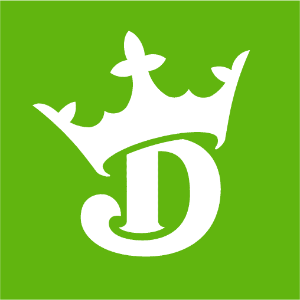DraftKings Super Bowl Odds
+500
5 to 1Ravens
13.6% implied probability

+500
5 to 1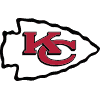Chiefs
13.6% implied probability

+1100
11 to 1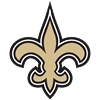Saints
6.8% implied probability

+1200
12 to 1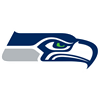Seahawks
6.3% implied probability

+1600
16 to 1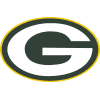Packers
4.8% implied probability

+1800
18 to 1Cowboys
4.3% implied probability

+1800
18 to 1Steelers
4.3% implied probability

+1800
18 to 1Buccaneers
4.3% implied probability

+2000
20 to 1Bills
3.9% implied probability

+2000
20 to 1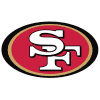49ers
3.9% implied probability

+2200
22 to 1Patriots
3.6% implied probability

+2200
22 to 1Rams
3.6% implied probability

+2500
25 to 1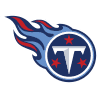Titans
3.1% implied probability

+2500
25 to 1Cardinals
3.1% implied probability

+3000
30 to 1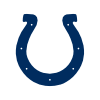Colts
2.6% implied probability

+3300
33 to 1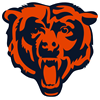Bears
2.4% implied probability

+4000
40 to 1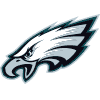Eagles
2.0% implied probability

+4000
40 to 1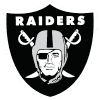Raiders
2.0% implied probability

+5000
50 to 1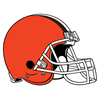Browns
1.6% implied probability

+5000
50 to 1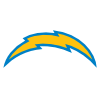Chargers
1.6% implied probability

+6000
60 to 1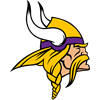Vikings
1.3% implied probability

+8000
80 to 1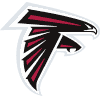Falcons
1.0% implied probability

+8000
80 to 1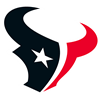Texans
1.0% implied probability

+10000
100 to 1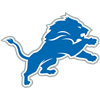Lions
0.8% implied probability

+10000
100 to 1Broncos
0.8% implied probability

+12500
125 to 1Football Team
0.6% implied probability

+15000
150 to 1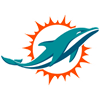Dolphins
0.5% implied probability

+15000
150 to 1Jaguars
0.5% implied probability

+15000
150 to 1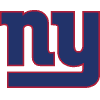Giants
0.5% implied probability

+20000
200 to 1Jets
0.4% implied probability

+20000
200 to 1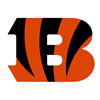Bengals
0.4% implied probability

+20000
200 to 1Panthers
0.4% implied probability